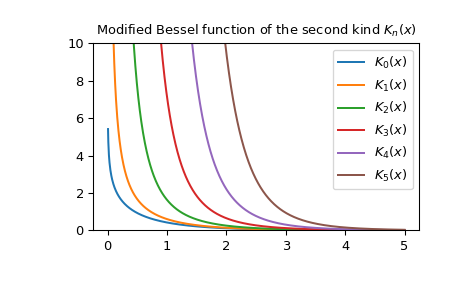# scipy.special.kn¶

scipy.special.kn(n, x) = <ufunc 'kn'>

Modified Bessel function of the second kind of integer order n

Returns the modified Bessel function of the second kind for integer order n at real z.

These are also sometimes called functions of the third kind, Basset functions, or Macdonald functions.

Parameters
narray_like of int

Order of Bessel functions (floats will truncate with a warning)

zarray_like of float

Argument at which to evaluate the Bessel functions

Returns
outndarray

The results

`kv`

Same function, but accepts real order and complex argument

`kvp`

Derivative of this function

Notes

Wrapper for AMOS  routine zbesk. For a discussion of the algorithm used, see  and the references therein.

References

1

Donald E. Amos, “AMOS, A Portable Package for Bessel Functions of a Complex Argument and Nonnegative Order”, http://netlib.org/amos/

2

Donald E. Amos, “Algorithm 644: A portable package for Bessel functions of a complex argument and nonnegative order”, ACM TOMS Vol. 12 Issue 3, Sept. 1986, p. 265

Examples

Plot the function of several orders for real input:

```>>> from scipy.special import kn
>>> import matplotlib.pyplot as plt
>>> x = np.linspace(0, 5, 1000)
>>> for N in range(6):
...     plt.plot(x, kn(N, x), label='\$K_{}(x)\$'.format(N))
>>> plt.ylim(0, 10)
>>> plt.legend()
>>> plt.title(r'Modified Bessel function of the second kind \$K_n(x)\$')
>>> plt.show()
```Calculate for a single value at multiple orders:

```>>> kn([4, 5, 6], 1)
array([   44.23241585,   360.9605896 ,  3653.83831186])
```